REDOX POTENTIALS FOR NON-METAL AND OTHER SYSTEMS

```
```

This page explains how non-metals like chlorine can be included in the electrochemical series, and how other oxidising and reducing agents can have their standard electrode potentials (redox potentials) measured and fitted into the series.

Important:  If you have come straight to this page via a search engine, you should be aware that this is just one page in a linked series of pages about redox potentials. You will find it much easier to understand if you start from the beginning. Links at the bottom of each page will bring you back here again.

It is also important that you understand about redox reactions. Follow this link if you aren't confident about oxidation and reduction in terms of electron transfer, and use the BACK button on your browser to return to this page.

Measuring redox potentials for more complicated systems

Systems involving gases

The obvious example here is chlorine. Chlorine is well known as an oxidising agent. Since the electrochemical series is about ranking substances according to their oxidising or reducing ability, it makes sense to include things like chlorine.

This time we are measuring the position of this equilibium relative to the hydrogen equilibrium.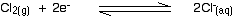Notice that the equilibrium is still written with the electrons on the left-hand side of the equation. That's why the chlorine gas has to appear on the left-hand side rather than on the right (which is where the metals and hydrogen appeared).

How can this equilibrium be connected into a circuit? The half-cell is built just the same as a hydrogen electrode. Chlorine gas is bubbled over a platinum electrode, which is immersed in a solution containing chloride ions with a concentration of 1 mol dm-3.

The conventional way of writing the whole cell looks like this.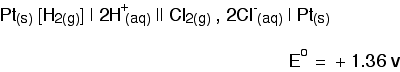Notice the way that the chlorine half cell is written. The convention is that the substance losing electrons is written closest to the electrode. In this case, the chloride ions are losing electrons.

Note:  Assuming that you know about oxidation states (oxidation numbers), that is exactly the same as saying that the substance with the lowest oxidation state is written closest to the electrode.

If you had the chlorine half cell on the left-hand side in a different situation, then the convention still has to hold. The half cell would then be written: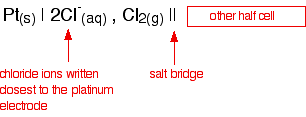What does the E° value show in the Cl2 / Cl - case?

The value is positive and moderately high as E° values go. That means that the position of the Cl2 / Cl - equilibrium lies more to the right than the hydrogen equilibrium. Chlorine is much more likely to pick up electrons than hydrogen ions are.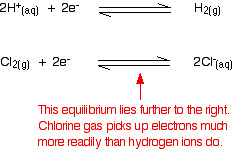Chlorine is therefore quite good a removing electrons from other things. It is a good oxidising agent.

```
```

Measuring redox potentials for other systems

The Fe2+ / Fe3+ system

Iron(II) ions are easily oxidised to iron(III) ions, and iron(III) ions are fairly easily reduced to iron(II) ions. The equilibrium we are interested in this time is: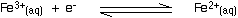To measure the redox potential of this, you would simply insert a platinum electrode into a beaker containing a solution containing both iron(II) and iron(III) ions (1 mol dm-3 with respect to both), and couple this to a hydrogen electrode.

The cell diagram would look like this:Notice that the E° value isn't as positive as the chlorine one. The position of the iron(III) / iron(II) equilibrium isn't as far to the right as the chlorine equilibrium. That means that Fe3+ ions don't pick up electrons as easily as chlorine does. Chlorine is a stronger oxidising agent than Fe3+ ions.

Potassium dichromate(VI) as an oxidising agent

A commonly used oxidising agent, especially in organic chemistry, is potassium dichromate(VI) solution acidified with dilute sulphuric acid. The potassium ions are just spectator ions and aren't involved in the equilibrium in any way.

The equilibrium is more complicated this time because it contains more things: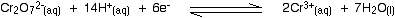The half cell would have a piece of platinum dipping into a solution containing all the ions (dichromate(VI) ions, hydrogen ions and chromium(III) ions) all at 1 mol dm-3.

There is yet another convention when it comes to writing these more complicated cell diagrams. Where there is more than one thing on either side of the equilibrium, square brackets are written around them to keep them tidy. The substances losing electrons are written next to the electrode, just as before.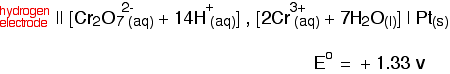```

```

Including these new redox potentials in the electrochemical series

These values can be slotted seamlessly into the electrochemical series that so far has only included metals and hydrogen.

An updated electrochemical series

equilibriumE° (volts)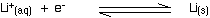-3.03-2.92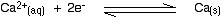-2.87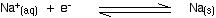-2.71-2.37-1.66-0.76-0.44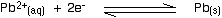-0.13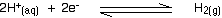0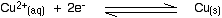+0.34+0.77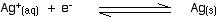+0.80+1.33+1.36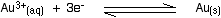+1.50

By coincidence, all the new equilibria we've looked at have positive E° values. It so happens that most of the equilibria with negative E° values that you meet at this level are ones involving simple metal / metal ion combinations.

An update on oxidising agents

Remember:

• The more positive the E° value, the further the position of equilibrium lies to the right.

• That means that the more positive the E° value, the more likely the substances on the left-hand side of the equations are to pick up electrons.

• A substance which picks up electrons from something else is an oxidising agent.

• The more positive the E° value, the stronger the substances on the left-hand side of the equation are as oxidising agents.

Of the new ones we've added to the electrochemical series:

• Chlorine gas is the strongest oxidising agent (E° = +1.36 v).

• A solution containing dichromate(VI) ions in acid is almost as strong an oxidising agent (E° = +1.33 v).

• Iron(III) ions are the weakest of the three new ones (E° = +0.77 v).

• None of these three are as strong an oxidising agent as Au3+ ions (E° = +1.50 v).

There will be more to say about this later in this series of pages.

```
```
 Questions to test your understanding If this is the first set of questions you have done, please read the introductory page before you start. You will need to use the BACK BUTTON on your browser to come back here afterwards. questions on redox potentials for other systems answers
```
```

Where would you like to go now?

To the next page on electrode potentials . . .

To the redox equilibria menu . . .

To the Physical Chemistry menu . . .

To Main Menu . . .

```
```

© Jim Clark 2002 (modified May 2013)